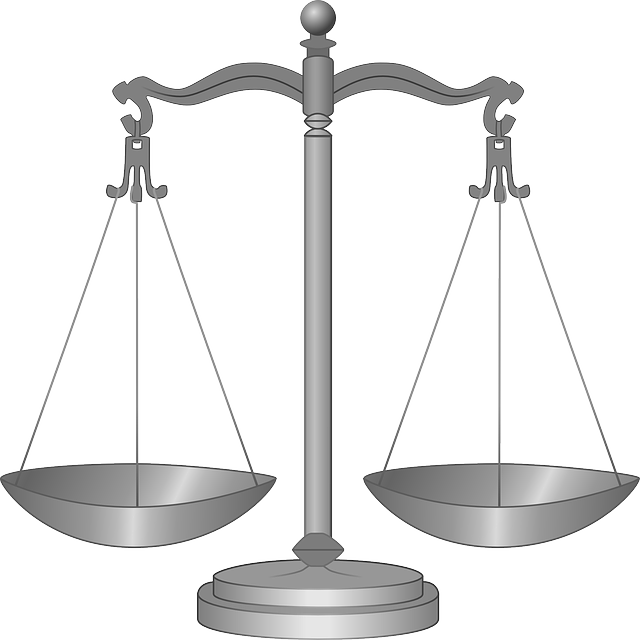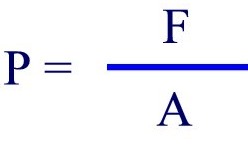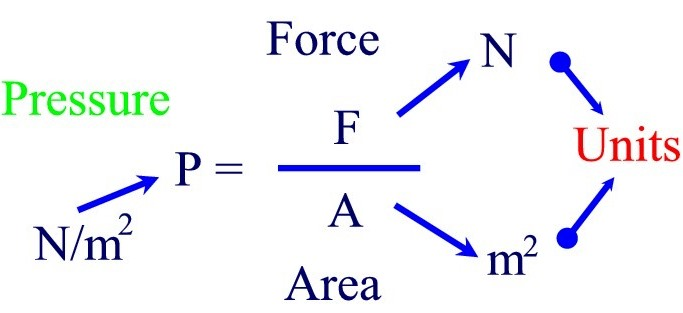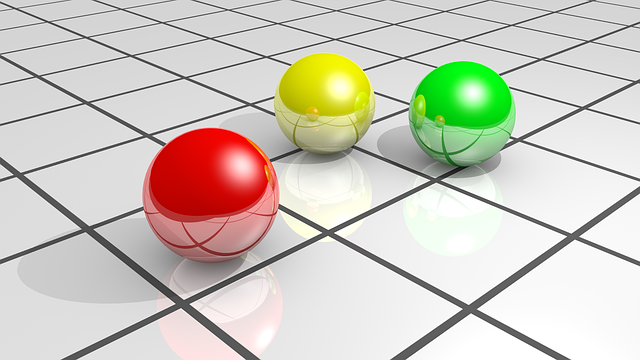#### Pressure

To find out what pressure is we carry out this simple activity:

• Gently press a pencil against the palm of you hand first using the tip of the pencil and then the back of the pencil, using the same amount of force in each case.
• What do you observe?

Observations: The tip of the pencil exerts the higer pressure, and there fore feels more uncomfortable against the skin than when the the back of the pencil is used with the same amount of "push".

Conclusion:  Under the same applied force, the smaller the area, the higher the pressure (pain)

Definifiton of Pressure: The scientific definition of Pressure is "the amount of force applied over a given area".  Pressure is directly proportional to the applied Force and inversely proportional to the Area.

Recall that force is defined as a push or a pull on an object.  Force is measured in Newtons with a spring scale.

Just to review a few important facts:

• The weight of an object is actually the force of gravity acting down on it, whereas the mass of the object is the amount of matter in it (all the atoms, and moleclues that make up the object).
• The Mass of an object is measured with a balance in grams, kilograms, and so on.
• To find out what the weight or Force of Gravity acting on an object with mass m, we multipy the mass by g (the acceleration due to gravity)
• the value of g on Earth is 9.8 N/KgThe images above show the different instruments used to measure force of gravity (spring scale) and mass (balance) respectively.  Note from the picture on the left, that the force of gravity on a 100 g (0.1Kg) medium sized apple is 1 Newton.

Let's continue with our definition of Pressure. As we said earlier, Pressure is defined as the Force per unit Area.

• The symbol for force is F,  measured in Newtons [N]
• the symbol for Area is A, measured in [m2 - squared meters],
• and Pressure has the symbol P.

Therefore, using the above symbols we can write this formula:Here is the complete expression with the respective units:Therefore, the units of pressure are N/m(Newtons per square meter).

The standard unit for pressure in the Metric System is the Pascal (pa), to honour Blaise Pascal,  a great french physicist and mathematician.

Note that 1  N/m2  = 1 Pa

However the "pascal" is a very small unit of pressure, therefore we usually measure pressure in a more convenient unit called the  Kpa - KiloPascal (1 Kpa = 1000 Pa).Example:

What is the pressure exerted by a 60 kg cannon ball resting on a 25 cm x 25 cm square floor tile?

Solution:

Given:

m = 60 Kg

g = 9.8 N/Kg

l = w = 25 cm

1. Calculate the Force in N.
The force in this case is the force of gravity on the child.

F = m x g

F = 60 Kg x 9.8 N/Kg

=  588 N

2. Calculate the Area

A = l x w

l = 25 cm = 0.25 m

w = 25 cm = 0.25 m

A = l x w = 0.25 m x 0.25 m

= 0.0625 m2

3. Calculate Pressure

P = F/A

= 588 N/0.0625 m2

Therefore, P = 9408 N/m2

P = 9408 Pa

= 9.4 KPa (2 digits accuracy)